# Game Theory (Normal-form Game) | Set 4 (Dominance Property-Pure Strategy)

In some of the games, it is possible to reduce the size of the payoff matrix by eliminating rows (or columns) which are dominated by other rows (or columns) respectively.

Dominance property for rows: X ≤ Y i.e. if all the elements of a particular row X are less than or equal to the corresponding elements of another row Y then delete row X (row X is dominated by row Y). The elements of a particular row X can also be compared with an average of two or more other rows and if the elements of the row X are less than or equal to the corresponding elements after taking the average then delete row X.

Dominance property for columns: X ≥ Y i.e. if all the elements of a particular column X are greater than or equal to the corresponding elements of another column Y then delete column X (column X is dominated by column Y). The elements of the column X can also be compared with an average of two or more columns and if the elements of column X are greater than the corresponding elements after taking the average then delete the column X.

Consider the below game: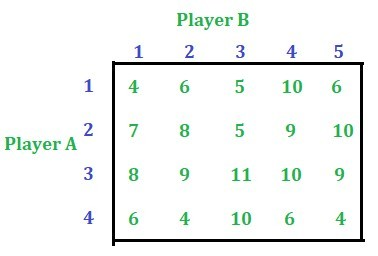Solution:
Pure Strategy: The solution of the above game by Pure Strategy will be,
– the value of the game (V) = 8
– A[P1, P2, P3, P4] = A[0, 0, 1, 0]
– B[Q1, Q2, Q3, Q4, Q5] = B[1, 0, 0, 0, 0]
Where,
P1, P2, P3 and P4 are probabilities of strategy 1, 2, 3 and 4 respectively for player A.
Q1, Q2, Q3, Q4 and Q5 are probabilities of strategy 1, 2, 3, 4 and 5 respectively for player B
For both the players, the total probability is 1.

Dominance Property: The same results will be obtained by using dominance property as well.
– Take the total of each row and select the least among them.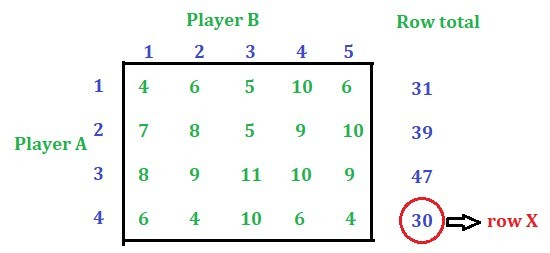– Using dominance property for row, elements of row 4 are smaller than the elements of row 3 i.e. row 4 is dominated by row 3. Delete row 4.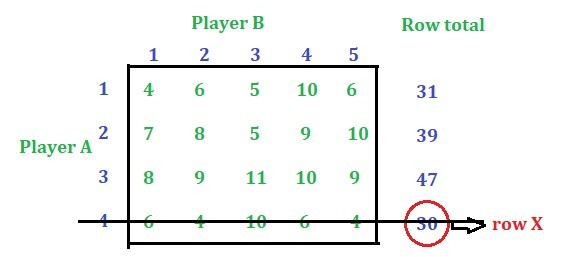– Select the least value among the remaining ‘Row total’ and apply the dominance property.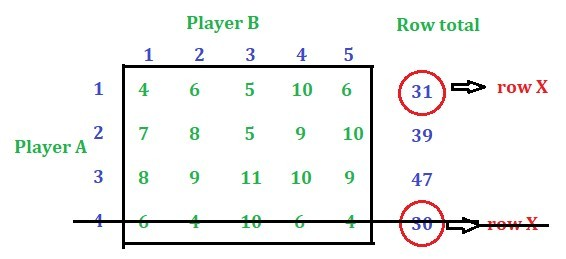– Using the dominance property, row 1 is dominated by row 3. Delete row 1.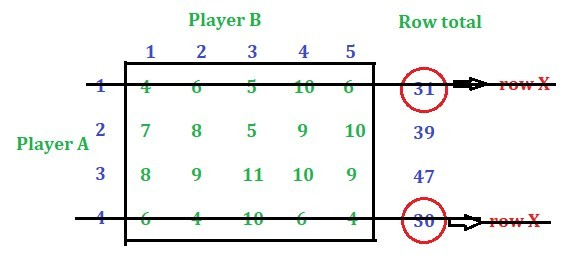– Select the least value among the remaining values i.e. 39 is the least, while applying the dominance property it can be seen that the condition for the row dominance is not satisfying. Now, apply the dominance property for column.

– Take the total of each column (only from the remaining rows) and select the largest among them.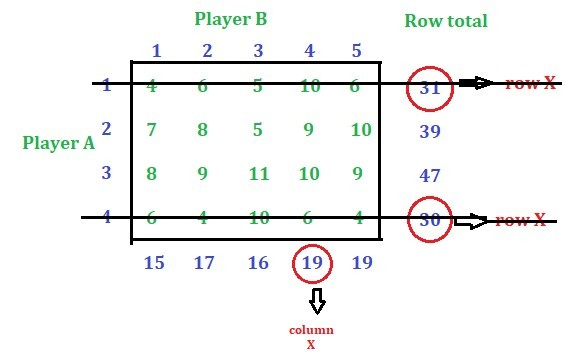– Using dominance property for column, column 4 is dominated by column 1. Delete the column 4.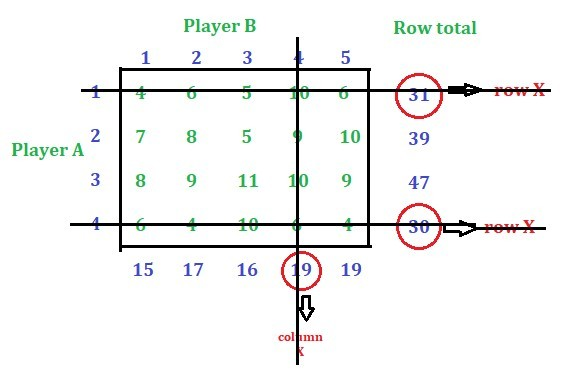– Select the largest among the reamining column values.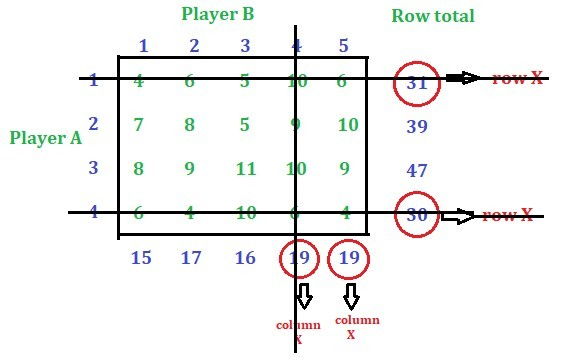– Using dominance property for columns, column 5 is dominated by column 1. Delete the column 5.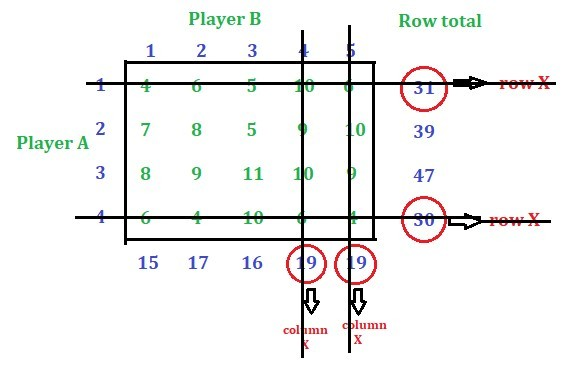– Select the largest among the remaining column values.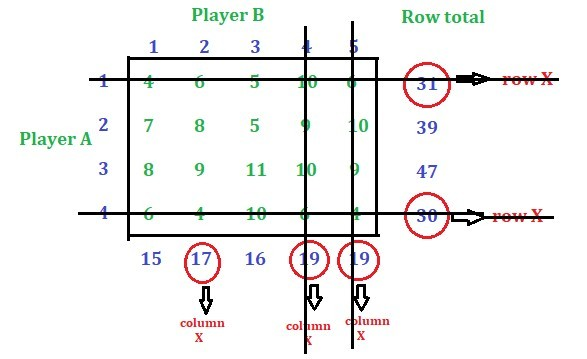– Using dominance property for columns, column 2 is dominated by column 1. Delete the column.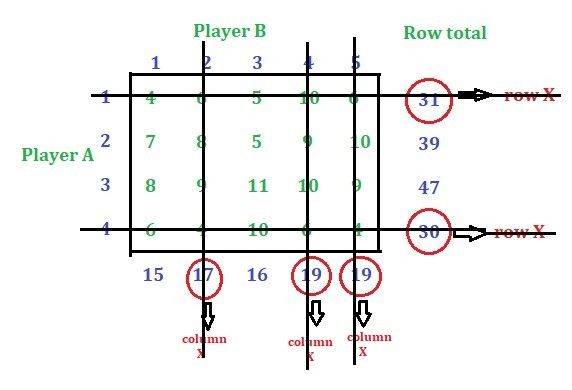– Again select the largest value (i.e. 16). Now, while applying the dominance property, no domination will be found. Now, again go for row reduction.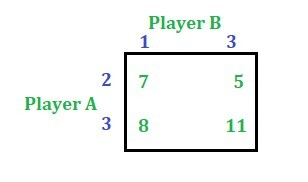– Find the row total and select the least among them.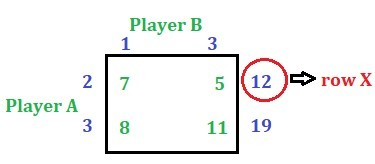– Using dominance property for rows, row 2 is dominated by row 3. Delete row 2.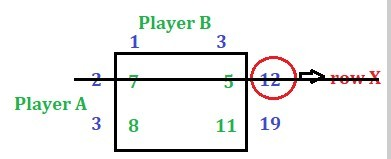– There is only one row now (No need to find the column total) and two columns remain. Applying the dominance property for columns.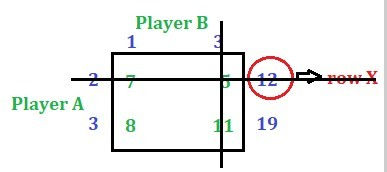– There is only one value remain which is the value of the game.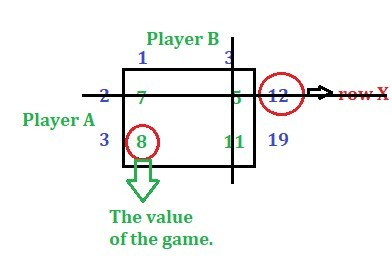So we have,
– the value of the game (V) = 8
– A[P1, P2, P3, P4] = A[0, 0, 1, 0]
– B[Q1, Q2, Q3, Q4, Q5] = B[1, 0, 0, 0, 0]
Where,
P1, P2, P3 and P4 are probabilities of strategy 1, 2, 3 and 4 respectively for player A.
Q1, Q2, Q3, Q4 and Q5 are probabilities of strategy 1, 2, 3, 4 and 5 respectively for player B
For both the players, the total probability is 1.

Attention reader! Don’t stop learning now. Get hold of all the important DSA concepts with the DSA Self Paced Course at a student-friendly price and become industry ready.

My Personal Notes arrow_drop_upCheck out this Author's contributed articles.

If you like GeeksforGeeks and would like to contribute, you can also write an article using contribute.geeksforgeeks.org or mail your article to contribute@geeksforgeeks.org. See your article appearing on the GeeksforGeeks main page and help other Geeks.

Please Improve this article if you find anything incorrect by clicking on the "Improve Article" button below.